# 5b.4: Proving the validity of immediate inferences

•• Andrew Lavin
• Butte College
$$\newcommand{\vecs}{\overset { \rightharpoonup} {\mathbf{#1}} }$$ $$\newcommand{\vecd}{\overset{-\!-\!\rightharpoonup}{\vphantom{a}\smash {#1}}}$$$$\newcommand{\id}{\mathrm{id}}$$ $$\newcommand{\Span}{\mathrm{span}}$$ $$\newcommand{\kernel}{\mathrm{null}\,}$$ $$\newcommand{\range}{\mathrm{range}\,}$$ $$\newcommand{\RealPart}{\mathrm{Re}}$$ $$\newcommand{\ImaginaryPart}{\mathrm{Im}}$$ $$\newcommand{\Argument}{\mathrm{Arg}}$$ $$\newcommand{\norm}{\| #1 \|}$$ $$\newcommand{\inner}{\langle #1, #2 \rangle}$$ $$\newcommand{\Span}{\mathrm{span}}$$ $$\newcommand{\id}{\mathrm{id}}$$ $$\newcommand{\Span}{\mathrm{span}}$$ $$\newcommand{\kernel}{\mathrm{null}\,}$$ $$\newcommand{\range}{\mathrm{range}\,}$$ $$\newcommand{\RealPart}{\mathrm{Re}}$$ $$\newcommand{\ImaginaryPart}{\mathrm{Im}}$$ $$\newcommand{\Argument}{\mathrm{Arg}}$$ $$\newcommand{\norm}{\| #1 \|}$$ $$\newcommand{\inner}{\langle #1, #2 \rangle}$$ $$\newcommand{\Span}{\mathrm{span}}$$$$\newcommand{\AA}{\unicode[.8,0]{x212B}}$$

Let’s take a second to look at the different immediate inferences using Venn diagrams. Why do they work? Why don’t the invalid inferences work?

## Square of Opposition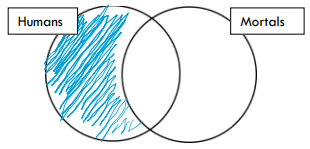Figure $$\PageIndex{1}$$: A: All humans are mortal thingsFigure $$\PageIndex{2}$$: O: Some humans are not mortal things

Taken together:There can’t be an X in a shaded area. We’ve “filled it with concrete” so there’s nothing there. What does this mean? These two propositions are claiming the opposite thing from one another. A is claiming that there are No things in region 1 (the left moon-shaped region), whereas O is claiming that there is at least one thing in region 1.Figure $$\PageIndex{3}$$: E: No humans are mortal thingsFigure $$\PageIndex{4}$$: I: Some humans are mortal things

Taken together:Again, E is claiming that there is nothing in region 2 (the ellipse or football shape in the center) and I is claiming that there’s at least one thing in region 2.

### ContrariesFigure $$\PageIndex{5}$$: A: All Humans are Mortal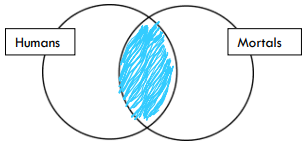Figure $$\PageIndex{6}$$: E: No Humans are Mortal

Taken together:Remember that these are only contrary on Aristotle’s assumption that the subject class has at least one member. Notice how in this diagram, the subject class (humans) must be empty. It is claiming that there are no humans. Since Aristotle assumes that the subject class is populated by at least one thing, it follows that A and E propositions cannot both be true (they are contraries).

I won’t do subcontraries or subalternation because they’re more complicated and hopefully you get the idea by now.

## Other Immediate Inferences

### Conversion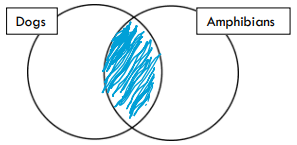Figure $$\PageIndex{7}$$: E: No Dog is an AmphibianFigure $$\PageIndex{8}$$: E*: No Amphibian is a Dog

See? They’re the same diagram! So the immediate inference is valid: we’re claiming the same thing in each proposition.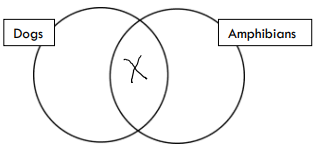Figure $$\PageIndex{9}$$: I: Some Dog is an AmphibianFigure $$\PageIndex{10}$$: I*: Some Amphibian is a Dog

Again, they look the same since they’re both claiming that there’s at least one thing in region 2.

But Conversion doesn’t work with A’s and O’s:Figure $$\PageIndex{11}$$: A: All Dogs are Amphibians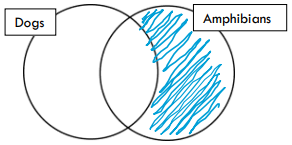Figure $$\PageIndex{12}$$: A*: All Amphibians are Dogs

Notice how they are claiming almost opposite things. At any rate, A* is certainly not contained in the diagram for A. (That’s what validity looks like, the conclusion is already in the diagram for the premises).Figure $$\PageIndex{13}$$: O: Some Dogs are not AmphibiansFigure $$\PageIndex{14}$$: O*: Some Amphibians are not Dogs

Again, they are claiming basically the opposite thing from one another and as a result, O* is not already represented in the diagram for O.

### Obversion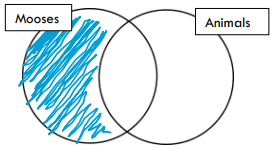Figure $$\PageIndex{15}$$: A: All Mooses (Meese?) are Animals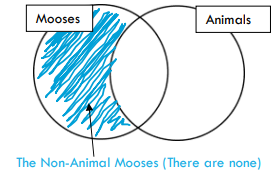Figure $$\PageIndex{16}$$: A*: No Mooses are Non-Animal

There are no mooses in the non-animal group, which is the left moon-shaped region, or region 1. So we shade it in.

All of the other Obversions end up with the same venn diagram too. Check it. Then give Contraposition a shot!

This page titled 5b.4: Proving the validity of immediate inferences is shared under a CC BY 4.0 license and was authored, remixed, and/or curated by Andrew Lavin via source content that was edited to the style and standards of the LibreTexts platform; a detailed edit history is available upon request.